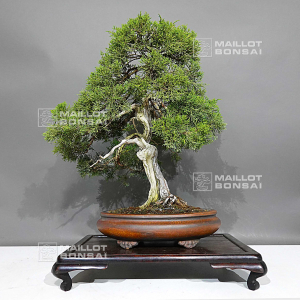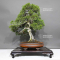##### The Japanese Bonsai specialist
Direct order Contact Help / Services Newsletter# Juniperus chinensis ref: 30080231

Juniperus chinensis itoigawaref. : 120291.880,00

voluminous/heavy item extra shipping of 12,00

Available quantity : 1Order

###### Description

Dimensions of the tree :
Height of the only tree : 540 mm.

Width of the branches : 480 mm.

Old hand-crafted quality pot : Dimensions : 340*260*85 mm.

Style : Moyogi.

Characterized by a large shari on its trunk and jins. Variety : Itoigawa.

A variety with particularly vigorous thin green scales that is perfectly adapted to grow as a boonsaï. Contrary to the standard juniperus chinensis variiety, it  keeps almost al its foliage in the beginning of summer.

Features: Well-formed bark, indicating the age of the tree (over 50 years old from yamadori). No significant injuries from wires or cutting. From the prestigious nurseries of Master Murakawa.
Authenticity: Genuine bonsai, featuring original jin and shari, not to be confused with imitations (tanuki).

The shari is exceptionally beautiful, giving this bonsai a unique character.

Recent Work: completely reworked in February 2020 by Master Tomoya Nishikawa, including cutting and the application of copper wires.

Deadwood has been lightly charred, adding an ashy effect that harmonizes with the overall appearance.

Photographed in August 2023.

The bonsai is sold without its stand. Feel free to admire this exceptional piece that embodies the beauty and history of the bonsai world.

#bonsai 6.6 #chinensis 3.9 #juniperus 3.9 #unique 3.6 #shari 3.5 #from 3.4 #with 3.4 #that 3.4 #tree 3.4 #dimensions 3

Formule
(( ROUND((CHAR_LENGTH(b.article_nom)-CHAR_LENGTH(REPLACE(b.article_nom, 'bonsai', '')))/LENGTH('bonsai')) + ROUND((CHAR_LENGTH(b.article_description)-CHAR_LENGTH(REPLACE(b.article_description, 'bonsai', '')))/LENGTH('bonsai')) ) * 4.6) + (( ROUND((CHAR_LENGTH(b.article_nom)-CHAR_LENGTH(REPLACE(b.article_nom, 'juniperus', '')))/LENGTH('juniperus')) + ROUND((CHAR_LENGTH(b.article_description)-CHAR_LENGTH(REPLACE(b.article_description, 'juniperus', '')))/LENGTH('juniperus')) ) * 3.9) + (( ROUND((CHAR_LENGTH(b.article_nom)-CHAR_LENGTH(REPLACE(b.article_nom, 'chinensis', '')))/LENGTH('chinensis')) + ROUND((CHAR_LENGTH(b.article_description)-CHAR_LENGTH(REPLACE(b.article_description, 'chinensis', '')))/LENGTH('chinensis')) ) * 3.9) + (( ROUND((CHAR_LENGTH(b.article_nom)-CHAR_LENGTH(REPLACE(b.article_nom, 'shari', '')))/LENGTH('shari')) + ROUND((CHAR_LENGTH(b.article_description)-CHAR_LENGTH(REPLACE(b.article_description, 'shari', '')))/LENGTH('shari')) ) * 3.5) + (( ROUND((CHAR_LENGTH(b.article_nom)-CHAR_LENGTH(REPLACE(b.article_nom, 'from', '')))/LENGTH('from')) + ROUND((CHAR_LENGTH(b.article_description)-CHAR_LENGTH(REPLACE(b.article_description, 'from', '')))/LENGTH('from')) ) * 3.4) + (( ROUND((CHAR_LENGTH(b.article_nom)-CHAR_LENGTH(REPLACE(b.article_nom, 'that', '')))/LENGTH('that')) + ROUND((CHAR_LENGTH(b.article_description)-CHAR_LENGTH(REPLACE(b.article_description, 'that', '')))/LENGTH('that')) ) * 3.4) + (( ROUND((CHAR_LENGTH(b.article_nom)-CHAR_LENGTH(REPLACE(b.article_nom, 'with', '')))/LENGTH('with')) + ROUND((CHAR_LENGTH(b.article_description)-CHAR_LENGTH(REPLACE(b.article_description, 'with', '')))/LENGTH('with')) ) * 3.4) + (( ROUND((CHAR_LENGTH(b.article_nom)-CHAR_LENGTH(REPLACE(b.article_nom, 'tree', '')))/LENGTH('tree')) + ROUND((CHAR_LENGTH(b.article_description)-CHAR_LENGTH(REPLACE(b.article_description, 'tree', '')))/LENGTH('tree')) ) * 3.4) + (( ROUND((CHAR_LENGTH(b.article_nom)-CHAR_LENGTH(REPLACE(b.article_nom, 'dimensions', '')))/LENGTH('dimensions')) + ROUND((CHAR_LENGTH(b.article_description)-CHAR_LENGTH(REPLACE(b.article_description, 'dimensions', '')))/LENGTH('dimensions')) ) * 3) + (( ROUND((CHAR_LENGTH(b.article_nom)-CHAR_LENGTH(REPLACE(b.article_nom, 'variety', '')))/LENGTH('variety')) + ROUND((CHAR_LENGTH(b.article_description)-CHAR_LENGTH(REPLACE(b.article_description, 'variety', '')))/LENGTH('variety')) ) * 2.7)

## Secure payment## Delivery

Our logistic partners :04 74 55 23 48
Pépinière MAILLOT-BONSAÏ
Le Bois Frazy
01990 RELEVANT - FRANCE
on appointment